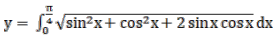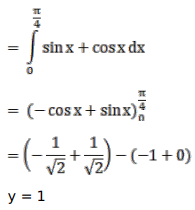# Mark against the correct answer in the following:

Question:

Mark $(\sqrt{ })$ against the correct answer in the following:

$\int_{0}^{\pi / 4} \sqrt{1+\sin 2 x} d x=?$

A. 0

B. 1

C. 2

D. $\sqrt{2}$

Solution: